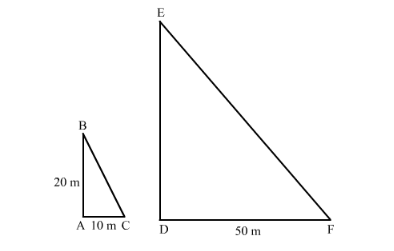Deepak Scored 45->99%ile with Bounce Back Crack Course. You can do it too!

# A vertical stick 20 m long casts a shadow 10 m long on the ground. At the same time, a tower casts a shadow 50 m long on the ground. The height of the tower is

Question:

A vertical stick 20 m long casts a shadow 10 m long on the ground. At the same time, a tower casts a shadow 50 m long on the ground. The height of the tower is

(a) $100 \mathrm{~m}$

(b) $120 \mathrm{~m}$

(c) $25 \mathrm{~m}$

(d) $200 \mathrm{~m}$

Solution:

Given: Vertical stick 20m long casts a shadow 10m long on the ground. At the same time a tower casts the shadow 50 m long on the ground.

To determine: Height of the tower

Let AB be the vertical stick and AC be its shadow. Also, let DE be the vertical tower and DF be its shadow.

Join BC and EF.In $\triangle \mathrm{ABC}$ and $\triangle \mathrm{DEF}$, we have

$\angle \mathrm{A}=\angle \mathrm{D}=90^{\circ}$

$\angle \mathrm{C}=\angle \mathrm{F}$

$\triangle \mathrm{ABC} \sim \triangle \mathrm{DEF}$

We know that in any two similar triangles, the corresponding sides are proportional. Hence,

$\frac{\mathrm{AB}}{\mathrm{DE}}=\frac{\mathrm{AC}}{\mathrm{DF}}$

$\frac{20}{\mathrm{DE}}=\frac{10}{50}$

$D E=100 \mathrm{~m}$

Hence the correct answer is option $(a)$.